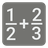Fractions Addition
Simple
English RU

Your task is to write a function which takes the tuple of tuples containing fractions as an argument and returns the sum of those fractions. The fractions will look like this: (x, y), where 'x' is the numerator, and 'y' is the denominator. For example, (2, 3) means 2/3. If the numerator is greater than the denominator (after the addition) you should extract the integer part and put it before the fraction. For example:
fractions (((2, 3), (2, 3))) = "1 and 1/3", because the result will be - 4/3 (the numerator is greater than the denominator) and you can extract the integer part (1) and the remaining fraction (1/3). Make note that the conjunction 'and' is required if the result has both parts - the integer and the fraction.
If the result doesn’t contain the fraction part and has only the integer - you should return it as the 'int'-type, not 'str'. If it doesn’t contain the integer part - just return it like a string 'N/D' where N - is the numerator and D - is the denominator.Input: Fractions.

Output: The sum of fractions.

Example:

```add_fractions(((2, 3), (2, 3))) == "1 and 1/3"
add_fractions(((1, 3), (1, 3))) == "2/3"
add_fractions(((1, 3), (1, 3), (1, 3))) == 1
```

How it is used: For work with non integer numbers.

Precondition :
2 <= the amount of the fractions <= 10
Positive fractions only.

Settings
Code:
CheckiO Extensions

CheckiO Extensions allow you to use local files to solve missions. More info in a blog post.

In order to install CheckiO client you'll need installed Python (version at least 3.8)

Install CheckiO Client first:

`pip3 install checkio_client`

`checkio --domain=py config --key=`

Sync solutions into your local folder

`checkio sync`

(in beta testing) Launch local server so your browser can use it and sync solution between local file end extension on the fly. (doesn't work for safari)

`checkio serv -d`

Alternatevly, you can install Chrome extension or FF addon

`checkio install-plugin`
`checkio install-plugin --ff`
`checkio install-plugin --chromium`

Read more here about other functionality that the checkio client provides. Feel free to submit an issue in case of any difficulties.

Sync...